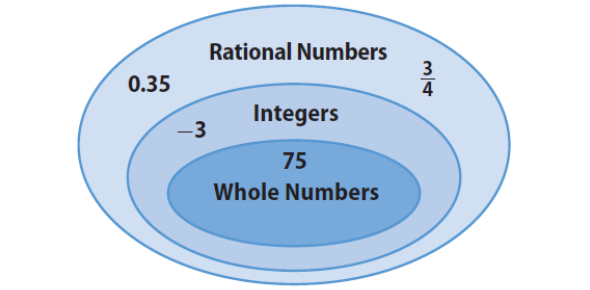# Integers And Set Of Real Numbers Quiz

10 Questions | Attempts: 6360
ShareSettingsAre you aware of integers, whole numbers, and sets of real numbers? Well then, why not test your knowledge with a quick, super fun quiz? Do you think you can answer all the questions without any help? If yes, then give our Math quiz a fair try. Please read the questions carefully before answering. All the questions are compulsory, and your marking will be dependent on the number of answers you get correct. Let's see if you can get a high score on this quiz! You can play this quiz as often as you like. Have fun!

• 1.
Identify the set(s) to which the number 21 belongs.
• A.

Natural numbers

• B.

Whole numbers

• C.

Integers

• D.

Rational numbers

• 2.
Identify the set(s) to which the number -15 belongs.
• A.

Natural numbers

• B.

Whole numbers

• C.

Integers

• D.

Rational

• 3.
Identify the set(s) to which the number 1/2 belongs.
• A.

Natural numbers

• B.

Whole numbers

• C.

Integers

• D.

Rational numbers

• 4.
Identify the set(s) to which the number 0 belongs.
• A.

Natural numbers

• B.

Whole numbers

• C.

Integers

• D.

Rational numbers

• 5.
Identify the set(s) to which the number -1/8 belongs.
• A.

Natural numbers

• B.

Whole numbers

• C.

Integers

• D.

Rational numbers

• 6.
Choose the rational number between 1 and 4.
• A.

2

• B.

3/2

• C.

3

• D.

1/2

• 7.
Choose the equation(s) that fit the pattern n/n = 1.
• A.

3/3 =1

• B.

1.5/1.5 =1

• C.

1,435/1,435 =1

• D.

0/0 = 1

• 8.
The number that can be written as a fraction is what?
• A.

Natural number

• B.

Rational number

• C.

Whole number

• D.

All of the above

• 9.
Which of the following does not apply to 7/8?
• A.

Integer

• B.

Rational number

• C.

Whole number

• D.

All of the above

• 10.
_________ is the set of numbers that includes positive and negative numbers.
• A.

Whole numbers

• B.

Rational numbers

• C.

Natural numbers

• D.

Integers

## Related TopicsBack to top
×

Wait!
Here's an interesting quiz for you.